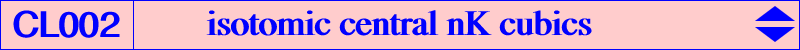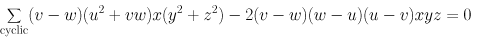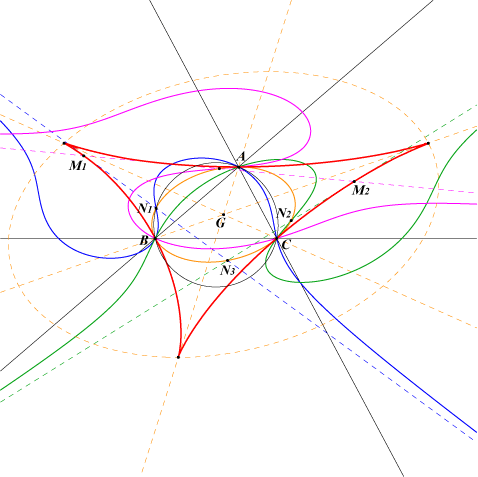All isotomic central nK have their center N on the Steiner circum-ellipse and they always have only one real asymptote since they all pass through the points where the Steiner ellipse meets the line at infinity. See a generalization in the page P-conical cubics. The "last" point at infinity is the isotomic conjugate N' of N. With N' = (u : v : w), the general equation of these cubics is :With N = X(99), we obtain the cubic K087. The real asymptote NN' envelopes a tricuspidal quartic passing through the infinite points of the Steiner ellipse counted twice. The contact M of NN' with the quartic is the reflection in N of the second intersection of NN' with the Steiner ellipse. If N1 and N2 are two antipodes on the Steiner ellipse the asymptotes concur on the Steiner ellipse at N3 and the midpoint of M1M2 is the reflection of N3 in G. The root R of the cubic is the complement of the isotomic conjugate of Z, trilinear pole of NN'. R lies on the homothetic of the Tucker nodal cubic K015 under h(G,1/4).